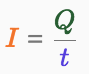### Electrical Current

Electrical current is the flow (movement) of electric charges. For an electric charge to flow there has to be a source of potential difference and a closed (or complete) circuit.

## Current

An electric current is the flow (movement) of electric charges. Electric current is measured in amperes (A). The flow of charge (C) = current (A) x time (s). A current has the same value at every point in a single closed loop.

The current is equal to the charge divided by the time.